## Solution to 1986 Problem 39

 We assume a relationship of the form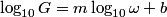\begin{align*} \log_{10} G = m \log_{10} \omega + b\end{align*}and attempt to calculate$m$. Raising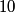$10$ to the power of both sides, we see that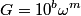$G = 10^b\omega^m$, so finding$m$ will allow us to distinguish between answers (B), (C), (D), and (E). From the two points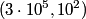$(3 \cdot 10^5, 10^2)$ and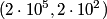$(2 \cdot 10^5, 2 \cdot 10^2)$, we find that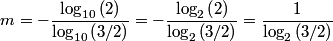\begin{align*}m = - \frac{\log_{10} \left(2\right)}{\log_{10} \left(3/2\right)} = - \frac{\log_{2} \left(2\right)}{\log_{2} \...Since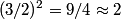$(3/2)^2 = 9/4 \approx 2$,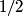$1/2$ provides a better approximation to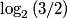$\log_2 \left(3/2\right)$ than$1$. Therefore,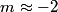\begin{align*}m \approx -2\end{align*}and answer (E) is correct.